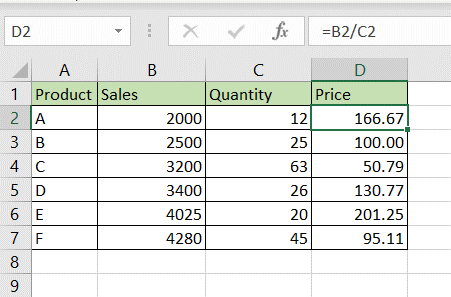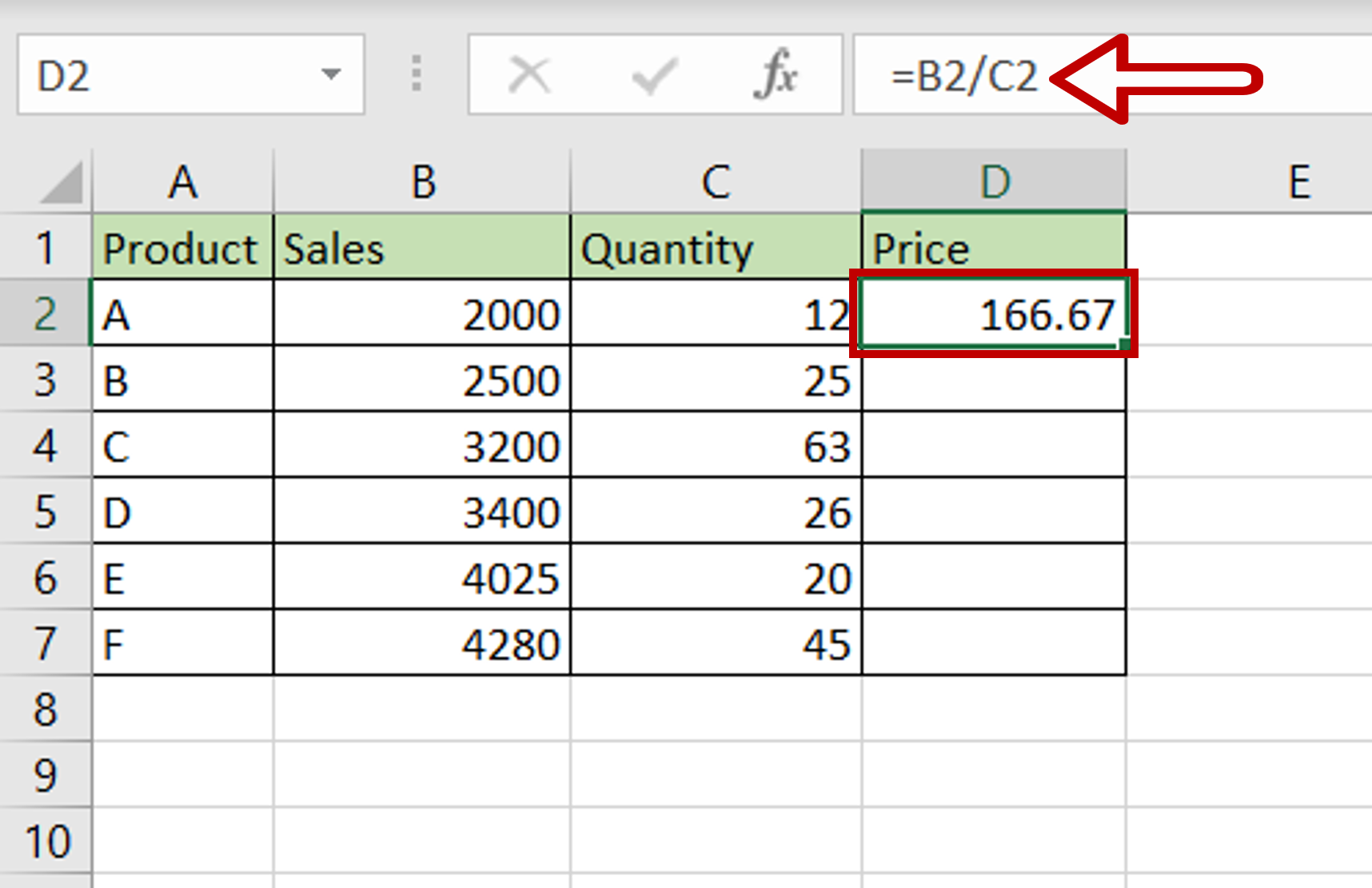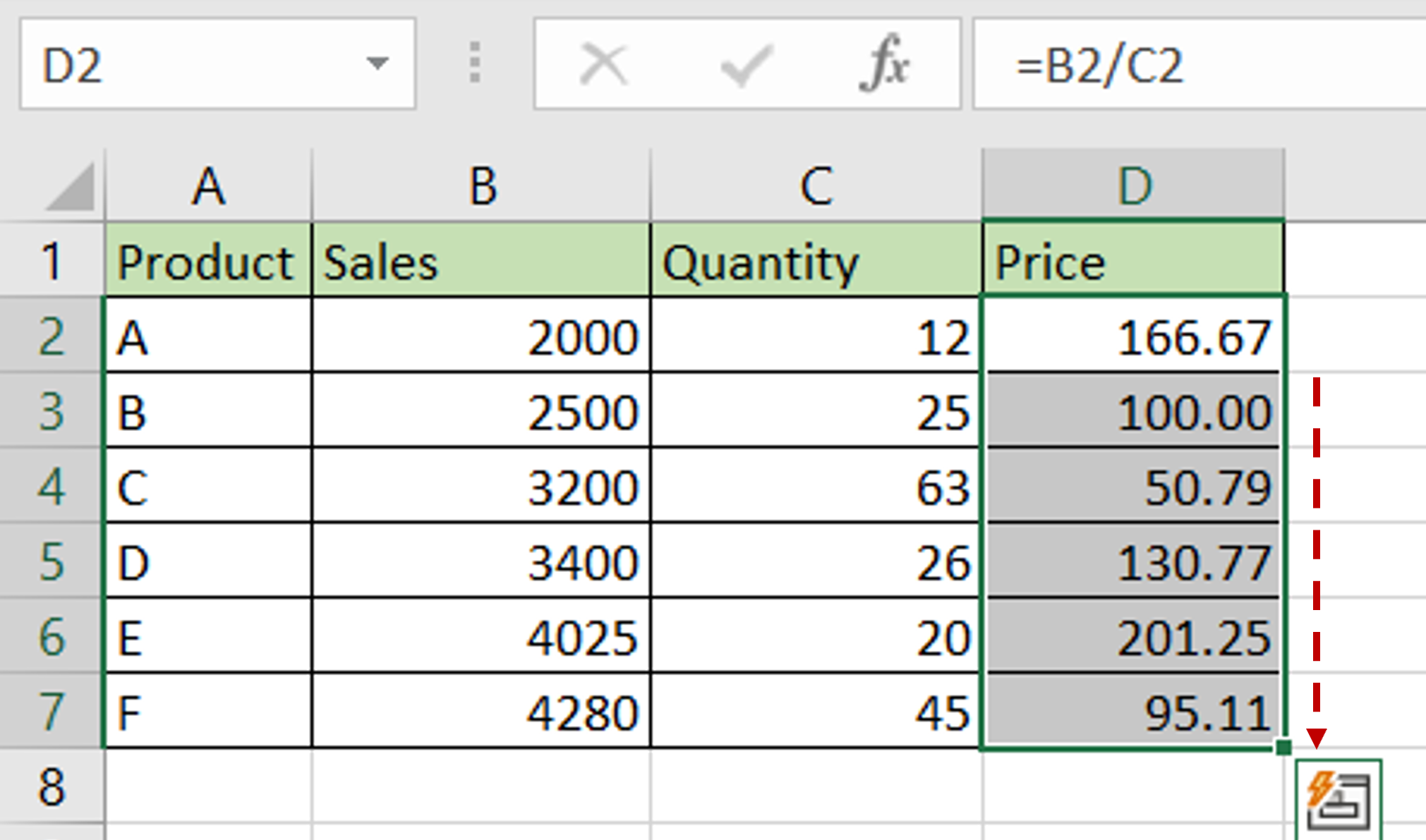# How to put a formula in Excel

You can watch a video tutorial here.One of the great things about Excel is the ability to create formulas that use cell references instead of actual values. A cell reference is the address of the cell and is made up of the column letter and the row number. As the row numbers and column letters are unique in Excel, each cell address is also unique. Using cell references in formulas introduces a lot of flexibility in calculations. Values in the source columns can be changed and the calculated columns will automatically be updated. Formulas created in one cell can be easily copied to other cells.

### Step 1 – Create the formula– Select the cell in which the formula is to be created
– Type the formula using cell references:
=Sales/Quantity
– Press Enter

### Step 2 – Copy the formula-Using the fill handle from the first cell, drag the formula to the remaining cells
OR
a) Select the cell with the formula and press Ctrl+C or choose Copy from the context menu (right-click)
b) Select the rest of the cells in the column and press Ctrl+V or choose Paste from the context menu (right-click)

### Step 3 – Check the result– Formulas are added to the column